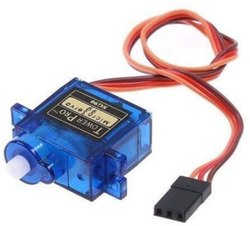In this project, we will see how to control servo motor with Arduino due

Summery

In this project, we will see how to control servo motor with Arduino due

Servo Motors:

We have used here SG90 Servo Motor. Servo Motors are recognized for their precise shaft movement or position. These are not offered for high-speed applications. It is having low speed, medium torque as well as precise position application. These motors are utilized in robotic arm machines, flight controls as well as control systems. These motors are also utilized in some printers and fax machines. It is available in various shapes and sizes.

Its three wires namely one is for positive voltage, second is for ground and the last one is for position setting. A Servo Motor is an integration of DC motor, position control system as well as gears. In servo, we have a control system that takes the PWM signal from the signal pin.It decodes the signal and acquires the duty ratio from it. After that, it equates the ratio to the values of the predefined position. If there is a variation in the values, it regulates the position of the servo accordingly. So the axis position of the servo motor relies on the duty ratio of the PWM signal to the SIGNAL pin.

#include <Servo.h>

Servo myservo;                 // providing a name

int angle = 0;                     // variable to store the servo position

void setup() {
myservo.attach(2);        // attaches the servo on pin 2 to the servo object
}

void loop() {
for (angle = 0; angle <= 180; angle += 1) {     // goes from 0 degrees to 180 degrees, in steps of 1 degree
myservo.write(angle);                                     // tell servo to go to position in variable 'angle'
delay(15);                                                        // waits 15ms for the servo to reach the position
}
for (angle = 180; angle >= 0; angle -= 1) {     // goes from 180 degrees to 0 degrees
myservo.write(angle);
delay(15);                                                       // waits 15ms for the servo to reach the position
}
}